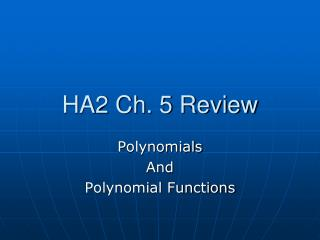DownloadDownload PresentationHA2 Ch. 5 Review

# HA2 Ch. 5 Review

Download Presentation## HA2 Ch. 5 Review

- - - - - - - - - - - - - - - - - - - - - - - - - - - E N D - - - - - - - - - - - - - - - - - - - - - - - - - - -
##### Presentation Transcript

1. HA2 Ch. 5 Review Polynomials And Polynomial Functions

2. Vocabulary • End behavior P.282 • Monomial P.280 • Multiplicity P. 291 • Polynomial Function P.280 • Relative Maximum/Min. P.291 • Standard Form of a Poly. Function • Synthetic Division P.306 • Turning Point P. 282

3. 5-1 Polynomial Functions • Degree of a Polynomial – highest exponent • Standard Form – in descending order • Define a polynomial by degree and by number of terms – See green table on P. 281 • Maximum # of Turning points: n-1 • End behavior – the far left and far right of the graph

4. Think in terms of a parabola If even, and a + Then end behavior upward facing End Behavior P. 282

5. Think in terms of a parabola If even, and a negative Then end behavior downward facing End Behavior

6. Think in terms of a parabola If ODD, and a + Then right end behavior upward facing, left is down End Behavior

7. Think in terms of a parabola If ODD, and a negative Then right end behavior downward facing, left is up End Behavior

8. Graphing Polynomial Functions • Step 1 – Find zeros and points in between. • Step 2 – “Sketch” graph • Step 3 – Use end behavior to check • Try to graph: y = 3x - x³ • Factored: 0 = x (3-x²) x = 0, ±√3

9. Graph

10. Assess • What is the end behavior and maximum amount of turning points in: (1.) y = -2x² - 3x + 3 (2.) y = x³ + x + 3

11. (1.) down and down, max 1 turning point (2.) down and up, max two tp

12. 5-2 Polynomials, Linear Factors, and Zeros • Factoring Polynomials: • GCF • Patterns: Diff of Squares, Perf. Sq. Trinomial • X-Method, Reverse Foil, Guess and Check • Set factors equal to zero and solve. • If those methods don’t work, then use Quadratic Formula to solve: • X =

13. Multiplicity – Factor repeats • What are the zeros of f (x) = x⁴ - 2x³ - 8x² and what are their mult. ? • = x (x² - 2x – 8) • = x (x + 2)(x – 4) • x = 0 (x2), -2, and 4

14. Writing a Function What is a cubic polynomial function in standard form with zeros 4, -1, and 2? y = (x – 4)(x + 1)(x – 2) y = (x² - 3x -4)(x – 2) y = x³ - 5x² + 2x + 8

15. 5-3 Solving Polynomial Equations • Two more patterns for factoring: • Sum/Diff of Cubes: • a³ + b³ = (a + b)(a² - ab +b) • a³ – b³ = (a - b)(a² + ab +b) • Factor completely: x³ - 27 • = (x – 3)(x + 3x + 9) • What are the real/imaginary solutions? • Solve: x = 3, ±

16. 5-3 Word Problem The width of a box is 2 m less than the length. The height is 1 m less than the length. The volume is 60 m³. What is the length of the box?

17. 5-4 Dividing Polynomials Remember to write in standard form and put a zero place holder. Use long division to determine if (x – 2) is a factor of x - 32. Remember in synthetic division if you have a fraction to divide your answer by denominator.